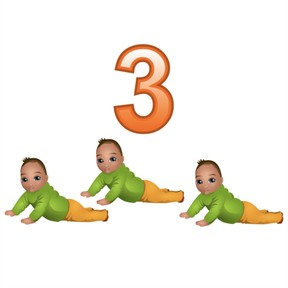Solving the 3 times table

# Solving the 3 times table

I can solve the times 3 multiplication facts.8,000 schools use Gynzy92,000 teachers use Gynzy1,600,000 students use Gynzy

## General

Students will learn the multiplication strategies for the times 3 facts. Instruction and practice will cover drawing arrays, skip counting, and adding one more group to a times two fact.

## Standards

CCSS.Math.Content.3.OA.B.5

## Learning objective

Students will be able to solve times 3 multiplication facts.

## Introduction

Students will discuss the meaning of “tri” as it relates to the number 3—the multiplication facts they will be learning.

## Instruction

The first strategy discussed is working with an array: objects in columns and rows to represent multiplication facts. Students will match arrays to multiplication facts. They will also shade them on graph paper. Next, students practice skip-counting the multiples of 3. This is followed by more fact practice. Students are then encouraged to build on what they know by using times 2 facts. For example, for 2 × 6 = 12, add one more group of 6 for 3 × 6 = 18. Students also review the commutative property as they complete another practice exercise.

## Quiz

Students respond to ten multiple-choice and true/false questions.

## Closing

Students will create story problems with answers that are multiples of 3. They will share these with a classmate.

## Instruction materials

To complete the story problem project, you will need:
- Construction paper
- something to color with

### The online teaching platform for interactive whiteboards and displays in schools

• Save time building lessons

• Manage the classroom more efficiently

• Increase student engagement